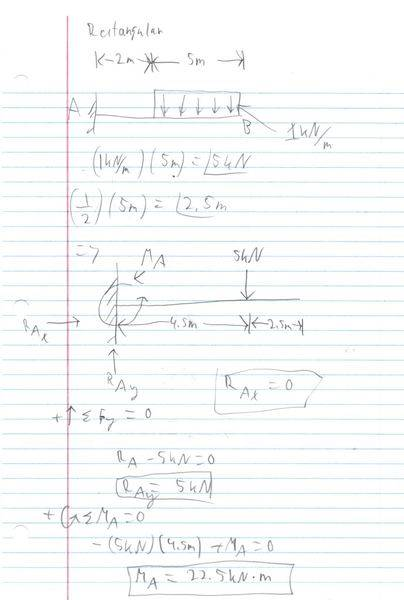# Calculating Shear and Bending forces in a Cantilever Beam.

## Homework Statement

I am trying to work out the Shear force and bending moments in a simple cantilever bean with a point load and a U.D.L but am not sure of the equations or how to go about starting it. Its a 2 m long cantilever beam built into a wall at the right-hand end, Point C. A UDL is applied with a downward force of 4 kN/m at Point B (which is 0.8 m from the free end) to the wall. An upward force of 2 kN is applied to the free (left-hand) end, Point A.

## Homework Equations

I presume you have to use equations for equilibrium but am not sure how to apply them.

## Homework Statement

I am trying to work out the Shear force and bending moments in a simple cantilever bean with a point load and a U.D.L but am not sure of the equations or how to go about starting it. Its a 2 m long cantilever beam built into a wall at the right-hand end, Point C. A UDL is applied with a downward force of 4 kN/m at Point B (which is 0.8 m from the free end) to the wall. An upward force of 2 kN is applied to the free (left-hand) end, Point A.

## Homework Equations

I presume you have to use equations for equilibrium but am not sure how to apply them.

## The Attempt at a Solution

first of all, you need to determine the reactions at the fixed end. what shape is the UDL? (rectangle, triangle etc). 4kn/m doesn't specify this.

a fixed end has 3 reactions. moment, force in y direction, force in x direction.

to find reactions, you need to sum the moments about a point (which will be equal to zero) then sum the forces in the y direction and the forces in the x direction. the sums will all be equal to zero. (you have no forces acting in the x direction, so just scratch that).

if you sum the moments about point A, you will have:

moment caused by shear force + moment caused by UDL + reaction moment of fixed end
support = 0

then

sum of forces in y direction:

- shear force - UDL + reaction y direction force of fixed end support = 0

notice the positive and negatives. the shear force and UDL are acting DOWN, the reaction of the fixed end has to COUNTERACT these forces so it points UP.

do you know how to find the moments about a point and how to find the points at which distributed loads act?

Am i righy in saying to find a moment you multiply the loads by the distance from the moment adding them togther and they should all = 0 (following sign convention).

To find the point at which the UDL acts you multiply the force by the length of the UDL and that force acts at the centre point of the UDL.

Am i righy in saying to find a moment you multiply the loads by the distance from the moment adding them togther and they should all = 0 (following sign convention).

To find the point at which the UDL acts you multiply the force by the length of the UDL and that force acts at the centre point of the UDL.

yes and no. it depends on what shape the UDL is. is it a rectangular shape? then yes, the UDL "acts" at the midpoint of the UDL. however, if the UDL is the shape of lets say a triangle, it "acts" 1/3 of the distance from the bigger end of the triangle. the SUM of all the forces in the y direction will equal zero, the SUM of all the forces in the x direction will equal zero, and the SUM of all the moments about a POINT will equal zero.

basically for a UDL, you find the area of the UDL, then that number is the total force it is inducing on the structure. You just have to find where its acting as a point load, then take the distance from where its acting to the point you're using as a reference (sum of moments about a point = 0) and multiply distance * force = moment. make sure you include all forces in the summations.

its easier if i just draw this real quick. heres an example of calculating the reactions of a fixed end cantilever beam with JUST a UDL. i won't do your work for you, but if you calculate your reactions and post back here ill help more.Last edited: